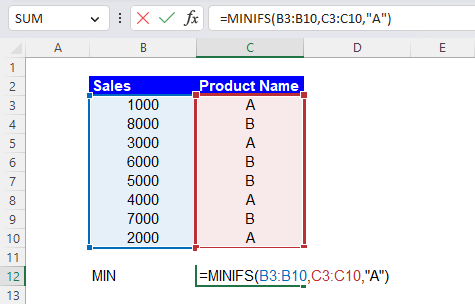Excel Formulas

# MINIFS Function in Excel: Explained

In this article, you will learn how to use the MINIFS formula in Excel.

## What is the MINIFS formula in Excel?

The MINIFS formula in Excel is a combination of the MIN and IF functions. The MINIFS formula returns the minimum value that meets one or more specified conditions.

## When do you need to use the MINIFS function in Excel?

The MINIFS function in Excel is useful when you need to find the minimum value from a range of cells that meet one or more specified requirements. Some examples of when you might use the MINIFS function include:

1. Finding the smallest sales amount from a list of sales, where the sales were made in a specific region and the salesperson's name is a specific person.
2. Finding the lowest score from a list of test scores, where the scores were earned by a specific grade level and the test was taken during a specific semester.
3. Finding the lowest temperature from a list of weather data, where the temperature was recorded during a specific month and at a specific time of day.

## How do you use the MINIFS formula in Excel?

The general syntax for the MINIFS formula is as follows:

``=MINIFS(min_range, criteria_range1, criteria1, [criteria_range2, criteria2], ...)``

min_range: The range of cells that you want to find the minimum value in.

criteria_range1: The range of cells that you want to apply the first condition to.

criteria1: The condition that you want to apply to criteria_range1.

criteria_range2, criteria2, ... [optional]: Additional ranges and conditions that you want to apply to find the minimum value. Additional criteria must be paired with the corresponding ranges.

Note: The size of the range or array for “min_range” and “criteria_rangeX” needs to be the same; otherwise the MINIFS formula returns the #VALUE! error value. Also note that if you enter text or date criteria manually in the function, don’t forget to enclose text with quotation marks.

Assume you have a list of sales figures in column B and a list of product names in column C. To find the highest sales figure for product "A", you would use the following formula: =MINIFS(B3:B10, C3:C10, "A")

In this example, the min_range is B3:B10, the criteria_range1 is C3:C10, and the criteria1 is "A". The formula returns 1,000, the smallest value among the sales with Product Name of A, which are 1,000, 3,000, 4,000, and 2,000.How to use the MINIFS function with a condition in Excel

Another example: Imagine you have a range of cells B16:B23 containing values and a range of cells C16:C23 containing criteria. You could use the following formula to find the minimum value in range B15:B22 where the corresponding value in C16:C23 is greater than 5.:

``=MINIFS(B16:B23, C16:C23, ">5")``

This formula returns 2,000 because it is the lowest value among the values with scores over 5, which are 8,000, 3,000, and 2,000.

You can also use multiple criteria like this example. Assume you have a list of sales figures in column B, a list of product names in column C, and a list of regions in column D. To find the smallest sales figure for product "A" and region "West", you would use the following formula:

``=MINIFS(B29:B36, C29:C36, "A", D29:D36, "West")``

In this example, the min_range is B29:B36, the criteria_range1 is C29:C36, the criteria1 is "A", the criteria_range2 is D29:D36, and the criteria2 is "West". The formula gives you 1,000, the minimum value among the sales with the Product Name of “A” in the “West” region, which are 1,000, 4,000, and 2,000.

## Why does the MINIFS function return zero in Excel?

The MINIFS function in Excel may return a zero if the range of cells that you are trying to find the minimum value in contains only zeroes or if the function fails to find any cells that meet the specified criteria. Here are some common reasons why the MINIFS function may return zero:

1. The min_range is empty or selected wrongly: The range of cells that you are trying to find the minimum value in contains only zeroes or blank cells or your range selection refer to a wrong range.
2. The criteria do not match any cells: If the conditions do not match any cells in the criteria_range, the function will return zero. Ensure that the standards match the data in the criteria_range, and that the requirements are in quotes if they are text.
3. The min_range contains only non-numeric values: If the range of cells in which you want to find the minimum value has only non-numeric values, the function will return zero.
4. The min_range contains only zero or negative numbers: If the range of cells in which you want to find the smallest value contains only zero or negative numbers, the function will return zero.

It's important to check these points and make sure that the range of cells that you are trying to find the minimum value in, and the criteria range and criteria are specified correctly in the MINIFS formula. Also, check the data to see if there are any errors or blank cells.

## Analyze your live financial data in a snap in Google Sheets

Are you learning this formula to visualize financial data, build a financial model, or conduct financial analysis? In that case, LiveFlow may help you automate manual workflows, update numbers in real-time, and save time. You can access various financial templates on our website, from the simple Income Statement to Multi-Currency Consolidated Financial Statement. Are you interested in this product but are an Excel user? That’s not a problem at all. You can connect Google Sheets to Excel quickly.